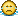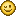### <Random float between 0.0 and 1.0> absolutely unnecessary!

#### alexsilent

Float Variables, how to create?
<Set [Speed] {Random number between <0.05> and <.1>}>
<Print [Speed]>
It's always Print "0"How I can create float variables?

Okay! I found {random float between 0.0 and 1.0} BUT it's a very bad way!
Because I need <0.05>-<0.1>, not <0.0>-<1.0>

« Last Edit: April 21, 2012, 02:08:18 pm by alexsilent »

#### captaincomic

You can use <0.5 + <random float between 0.0 and 1.0> / 2> to get a random number between 0.5 and 1.

In general
<Min + <random float between 0.0 and 1.0> * <Max - Min>> will give you a random number between Min and Max.

#### alexsilent

You can use <0.5 + <random float between 0.0 and 1.0> / 2> to get a random number between 0.5 and 1.

In general
<Min + <random float between 0.0 and 1.0> * <Max - Min>> will give you a random number between Min and Max.

Thanks, captaincomic! I found more easy way:
{<Random number between <1> and <100>> * <.01>}
There is only ONE mathematical action. It's like {Random Float <.01> and <1.0>}
Then It's more fastest.

other Example:
{<Random number between <-555> and <555>> * <.001>}
it's like -> {Random Float <-.555> and <.555>}

Then, <random float between 0.0 and 1.0> absolutely unnecessary.

« Last Edit: April 21, 2012, 02:10:47 pm by alexsilent »

#### captaincomic

It's not exactly the same.
<random number between <0> and <100>> * <0.01>
Will return for example: 0.03, 0.42, or 0,99.

While
<random float between 0.0 and 1.0>
will return: 0.03427865789023, 0.424778367856378, or 0,991467836482.

(I didn't count the number of decimal places, but you get the point)

#### ShivaFang

captaincomic is right, it is about percision level.  Random number between x and y returns only an integer.

Actually random number between x and y probably generates a random float betweeon 0.0 and 1.0, then multiplies by y-x, then adds x and truncnicates.  Result = Trunc((Rand()*(y-x))+x) <- this is how 'real' programmers need to do it in most other programming environments.  So using Random Number between X and Y and then multiplying like you suggest is actually NOT one mathematical action.  Stencyl just hides the math behind how the computer needs to do it.

Basically, random float between 0.0 and 1.0 is your 'basic' randomizer, wheras random number between X and Y is an 'easymode' wrapper for how most people want to generate random numbers.

Random Float is still imporatnt to most of us who have dealt a lot with random number generators, or require higher precision floats.
Justin "ShivaFang" White
Aquamentos Games - The origin of challenging Strategy and Role-Playing Flash gaming!